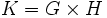# Normal subdirect product of perfect groups equals direct product

## Statement

Suppose$G$ and$H$ are Perfect group (?)s. Then, if$K$ is a Subdirect product (?) of$G$ and$H$ such that$K$ is normal inside$G \times H$, then$K = G \times H$.

## Proof

Given: Perfect groups$G,H$, with projections$p_1:G \times H \to G, p_2:G \times H \to H$. A normal subgroup$K$ of$G \times H$ such that$p_1(K) = G, p_2(K) = H$.

To prove:$K = G \times H$.

Proof: View$G$ and$H$ as subgroups in$G \times H$ by the embeddings as$G \times \{ e \}$ and$\{ e \} \times H$ respectively.

Since$G$ is normal in$G \times H$,$[K,G] \le G$. In particular,$p_1([K,G]) = [K,G]$.

Further,$p_1([K,G]) = [p_1(K),p_1(G)] = [G,G] = G$. Thus$[K,G] = G$.

But$K$ was assumed to be normal in$G \times H$, so$[K,G] \le K$. Thus,$G \le K$.

A similar argument shows that$H \le K$, so$K$ contains both$G$ and$H$. Hence,$K = G \times H$.